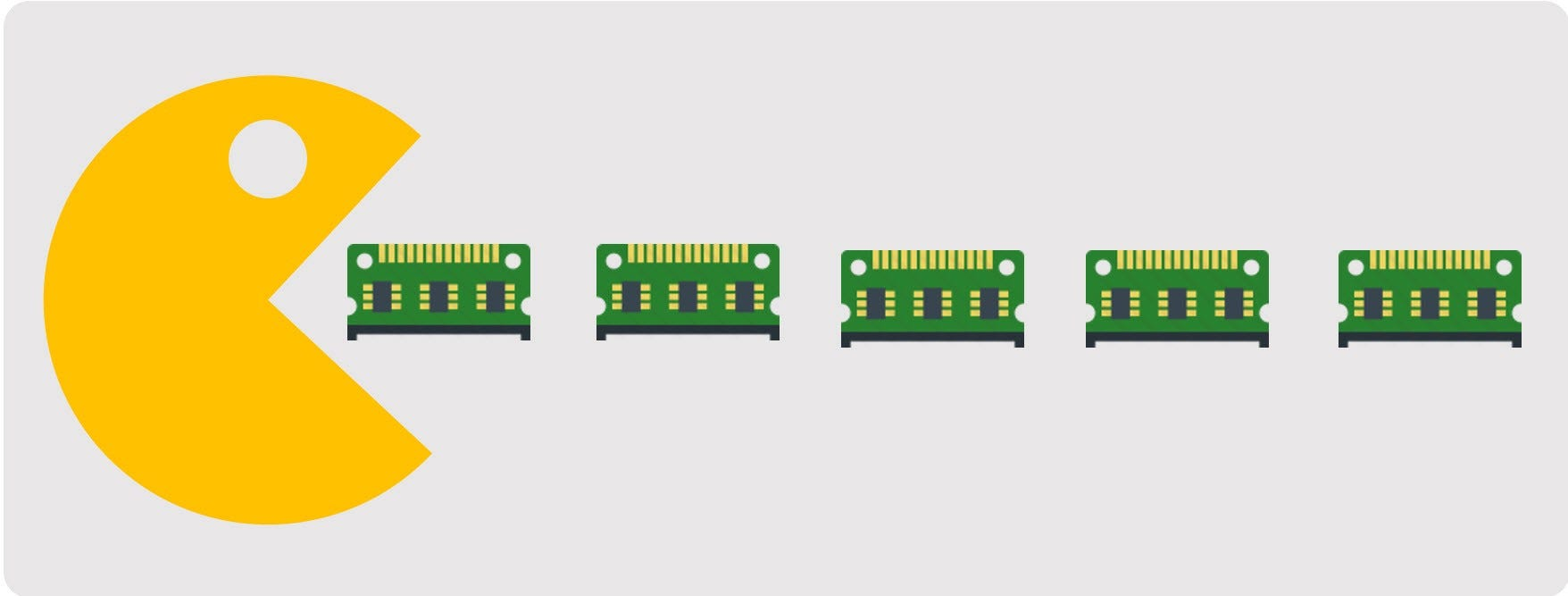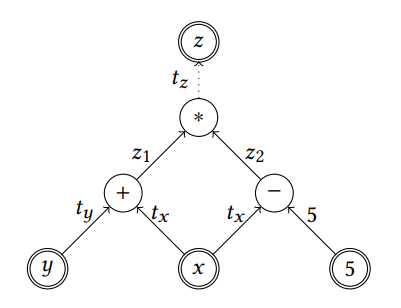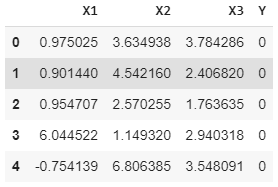# Contents(Source: https://miro.medium.com/max/3512/1*d-ZbdImPx4zRW0zK4QL49w.jpeg)

# Deep Learning Analysis Using Large Model Support

## Optimize your Machine Learning model memory consumption with IBM Large Model Support.

### Introduction

Memory management is now a really important topic in Machine Learning. Because of memory constraints, it is becoming quite common to train Deep Learning models using cloud tools such as Kaggle and Google Colab thanks to their free NVIDIA Graphical Processing Unit (GPU) support. Nonetheless, memory can still be a huge constraint in the cloud when working with large amounts of data.

In my last article, I explained how to speed up Machine Learning workflow execution. This article aims instead to explain to you how to efficiently reduce memory usage when implementing Deep Learning models. In this way, you might be able to train your Deep Learning model using the same amount of memory (even if before you couldn’t because of memory errors).

There are three main reasons for which a model can lead to running out of memory:

• Model depth/complexity = number of layers and nodes in a Neural Network.
• Data Size = number of samples/features in the dataset used.
• Batch Size = number of samples that get propagated through a Neural Network.

One solution to this problem has traditionally been to reduce the model size by trying to get rid of less relevant features during the preprocessing stage. This can be done using either Feature Importance or Feature Extraction techniques (eg. PCA, LDA).

Using this approach can possibly lead to reduced noise (decreasing the chance of overfitting) and faster training times. One downside though to this approach can be a consistent decrease in accuracy.

If the model needs a high complexity to capture all the important characteristics of a dataset, reducing the dataset size will in fact inevitably lead to worse performances. In this case, Large Model Support can be the solution to this problem.

### Large Model Support

Large Model Support (LMS) is a Python library recently launched by IBM. This library has been ideated in order to train large Deep Learning models which can’t fit in GPU memory. In fact, GPUs have generally smaller memory space compared to Central Processing Units (CPUs).

When Neural Networks are implemented using libraries such as Tensorflow and PyTorch, a set of mathematical operations gets automatically generated to construct this model. These mathematical operations can then be represented using computational graphs.

A computational graph is a directed graph where the nodes correspond to operations or variables. Variables can feed their value into operations, and operations can feed their output into other operations. This way, every node in the graph defines a function of the variables.

— deep ideas 

The values that enter and comes out of nodes in computational graphs are called tensors (multi-dimensional arrays).

In Figure 1 is represented a simple example of how a mathematical operation can be represented using a computational graph ( z = (x + y) ∗ (x − 5) ):Figure 1: Computational Graph 

LMS is able to levitate GPUs memory problems by redesigning Neural Networks computational graphs. This is done by making possible to transfer tensor operations by storing intermediate results on CPUs (instead of GPUs).

The IBM documentation outlines three different methods to use Large Model Support using the Tensorflow library:

• Session-based training.
• Estimator-based training.
• Keras-based training.

In this article, I will provide an example using Keras-based training. If you are interested in finding out more about the two other methods, IBM documentation is a great place where to start .

When working with LMS there are two main parameters we can tune in order to improve our model efficiency. The objective is to be able to find out the minimum number of tensors we need to swap out without incurring in memory errors.

The two main parameters to tune are:

• n_tensors = number of swapped tensors (eg. swiping out more tensors than needed, can lead to communication overheads).
• lb = how soon tensors are swapped back in before use (eg. using a low value for lb can make GPU training pause).

### Demonstration

I will now walk you through a simple example to get you started with LMS. All the code used for this exercise is available in this Google Colaboratory notebook and on my GitHub.

In this example, I will train a simple Neural Network using first Keras with Large Model Support and then just plain Keras. I both cases, I will record the memory usage required for the training.

#### Preprocessing

In order to install all the required dependencies to follow this example, just run the following cell in your notebook and enable your GPU environment (eg. Kaggle, Google Colab).

``````! git clone
! pip install ./tensorflow-large-model-support
! pip install memory_profiler
``````

Once is everything set up, we can then import all the necessary libraries.

In order to record the memory usage, I decided to use Python memory_profiler.

Successively, I defined the LMS Keras Callback which will be used during training. The definition of a Callback according to Keras documentation is:

A callback is a set of functions to be applied at given stages of the training procedure. You can use callbacks to get a view on internal states and statistics of the model during training.

— Keras Documentation 

Callbacks are typically used to take control of a model training process by automating certain tasks during every training iteration (in this case by adding Large Model Support optimization).

I then decided to fabricate a simple dataset of 200000 rows using Gaussian Distributions consisting of three features and two labels (0/1).

The values of the means and standard deviations of the distributions have been chosen so that to make this classification problem fairly easy (linearly separable data).Once created the dataset, I divided it into features and labels and then defined a function to preprocess it.

Now that we got our Training/Test sets, we are finally ready to get started with Deep Learning. I, therefore, defined a simple Sequential model for binary classification and selected a batch size of 8 elements.

#### Keras and Large Model Support

When using LMS, a Keras model is trained using Keras fit_generator function. The first input this function needs, is a generator. A generator is a function used to generate a dataset on multiple cores in real-time and then input its results in a Deep Learning model .

In order to create the generator function used in this example, I took reference to this implementation.

In case you are interested in a more detailed explanation about Keras Generators, it is available here.

Once defined our generator function I then trained our model using the LMS Keras Callback defined before.

In the code above, I additionally added on the first line the *%%memit *command to print out the memory usage of running this cell. The results are shown below:

``````Epoch 1/2 200000/200000 [==============================]
- 601s 3ms/step - loss: 0.0222 - acc: 0.9984

Epoch 2/2 200000/200000 [==============================]
- 596s 3ms/step - loss: 0.0203 - acc: 0.9984

peak memory: 2834.80 MiB, increment: 2.88 MiB
``````

The registered Peak Memory to train this model using LMS was equal to 2.83GB and the Increment 2.8MB.

Finally, I decided to test the accuracy of our trained model to validate our training results.

``````Model accuracy using Large Model Support: 99.9995 %
``````

#### Keras

Repeating the same procedure using plain Keras, the following results were obtained:

``````Epoch 1/2 1600000/1600000 [==============================]
- 537s 336us/step - loss: 0.0449 - acc: 0.9846

Epoch 2/2 1600000/1600000 [==============================]
- 538s 336us/step - loss: 0.0403 - acc: 0.9857

peak memory: 2862.26 MiB, increment: 26.15 MiB
``````

The registered Peak Memory to train this model using Keras was equal to 2.86GB and the Increment 26.15MB.

``````Model accuracy using Sklearn: 98.4795 %
``````

### Evaluation

Comparing the results obtained using *Keras + LMS vs Plain Keras *it can be noticed that using LMS can lead to a decrease in memory consumption and as well to an increase in model accuracy. LMS performance can even be even improved if given more GPU/CPU resources (which can be used to optimise training) and using larger datasets.

### Bibliography

 Deep Learning From Scratch I: Computational Graphs — deep ideas. Accessed at: http://www.deepideas.net/deep-learning-from-scratch-i-computational-graphs/

 TFLMS: Large Model Support in TensorFlow by Graph Rewriting. Tung D. Le, Haruki Imai et al. Accessed at: https://arxiv.org/pdf/1807.02037.pdf

 Getting started with TensorFlow Large Model Support (TFLMS) — IBM Knowledge Center. Accessed at: https://www.ibm.com/support/knowledgecenter/en/SS5SF7_1.5.4/navigation/pai_tflms.html

 Kears Documentation. Docs -> Callbacks. Accessed at: https://keras.io/callbacks/?source=post_page—————————

 A detailed example of how to use data generators with Keras.
Shervine Amidi. Accessed at: https://stanford.edu/~shervine/blog/keras-how-to-generate-data-on-the-fly

## Contacts

If you want to keep updated with my latest articles and projects follow me on Medium and subscribe to my mailing list. These are some of my contacts details:## Subscribe to my Data Blog Newsletter to get news about Data, Cloud and my website!

* indicates required